Examples

Chapter 13 Class 8 Introduction to Graphs
Serial order wise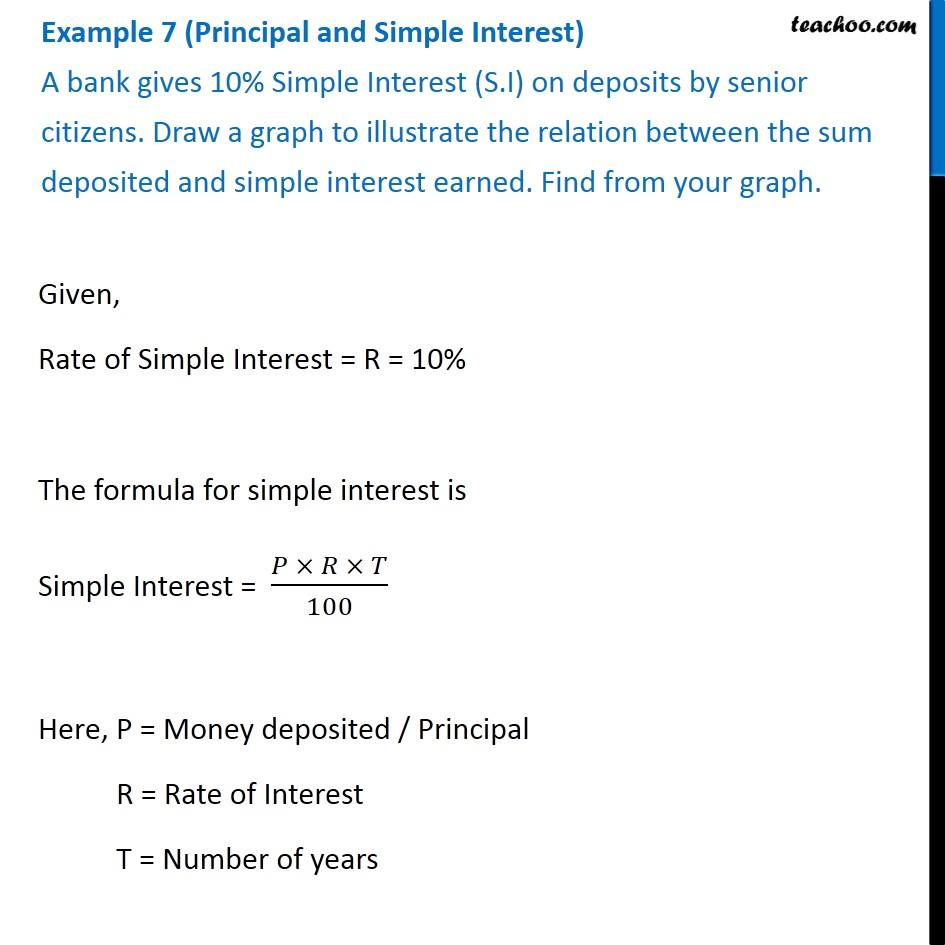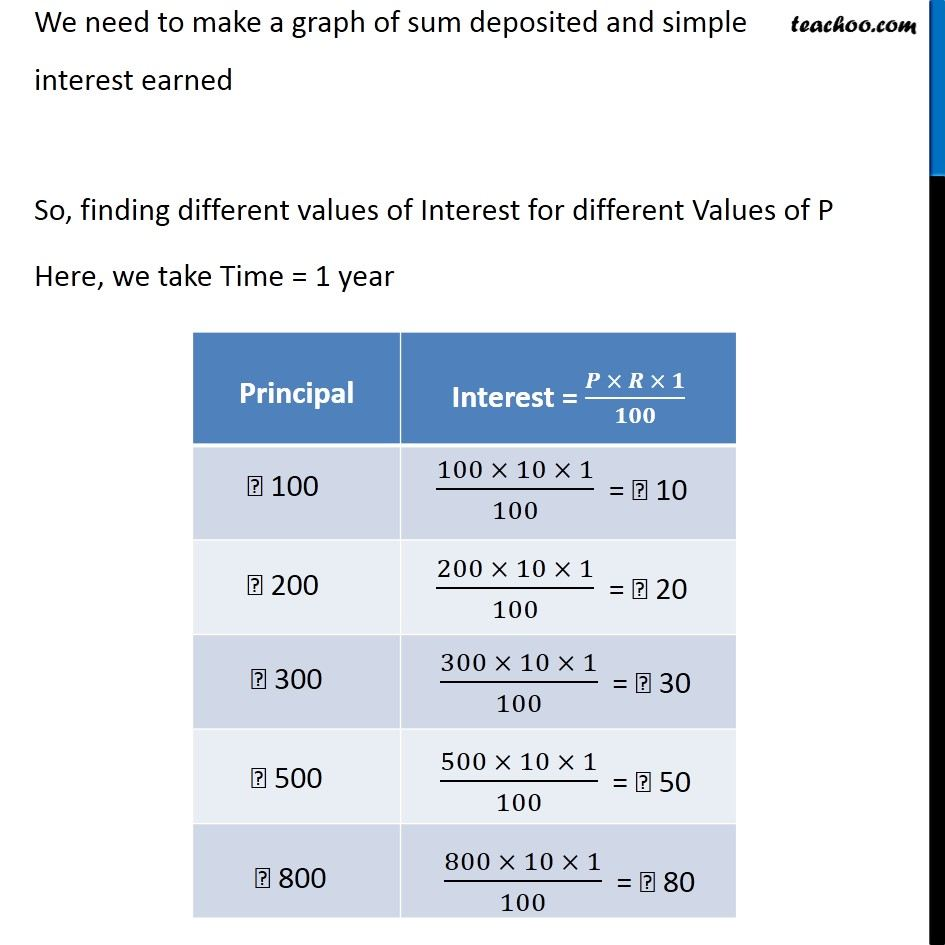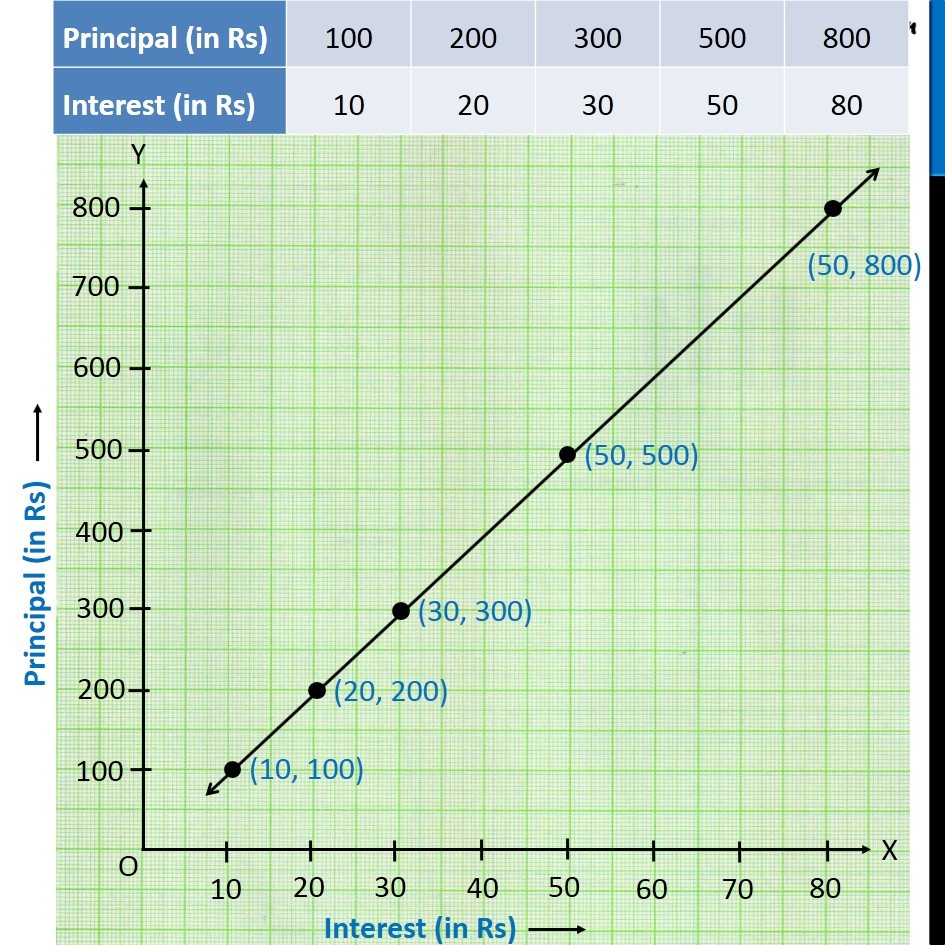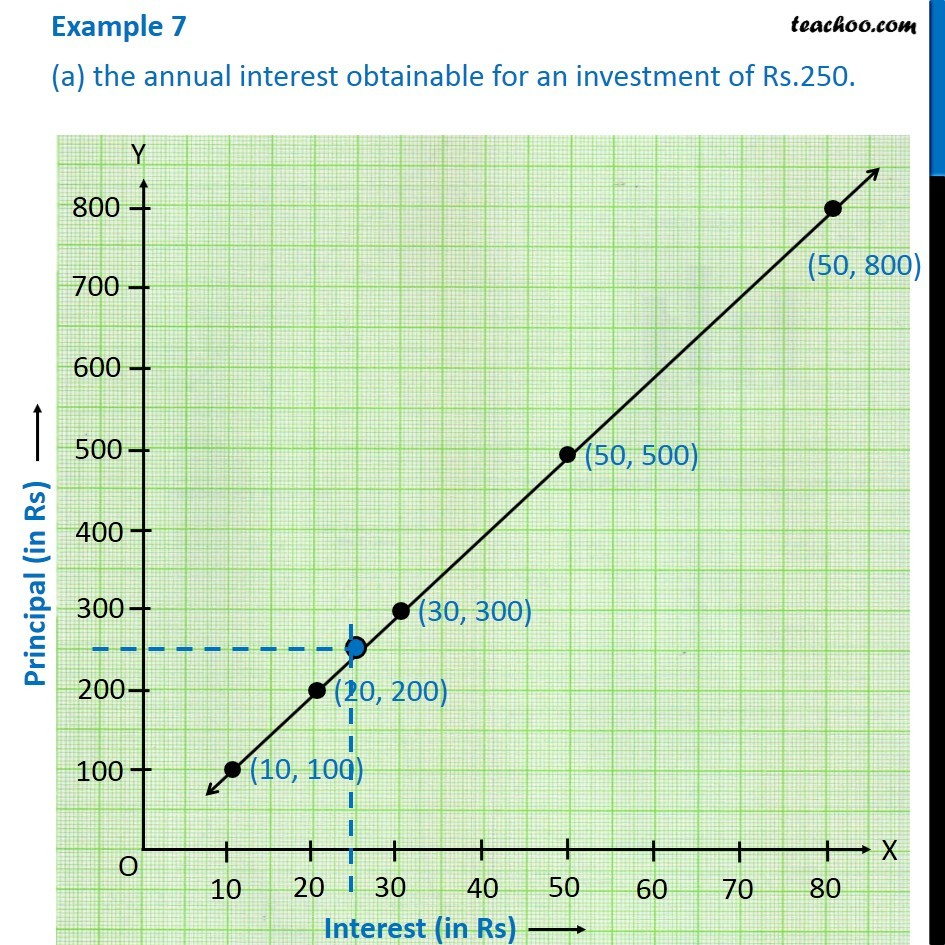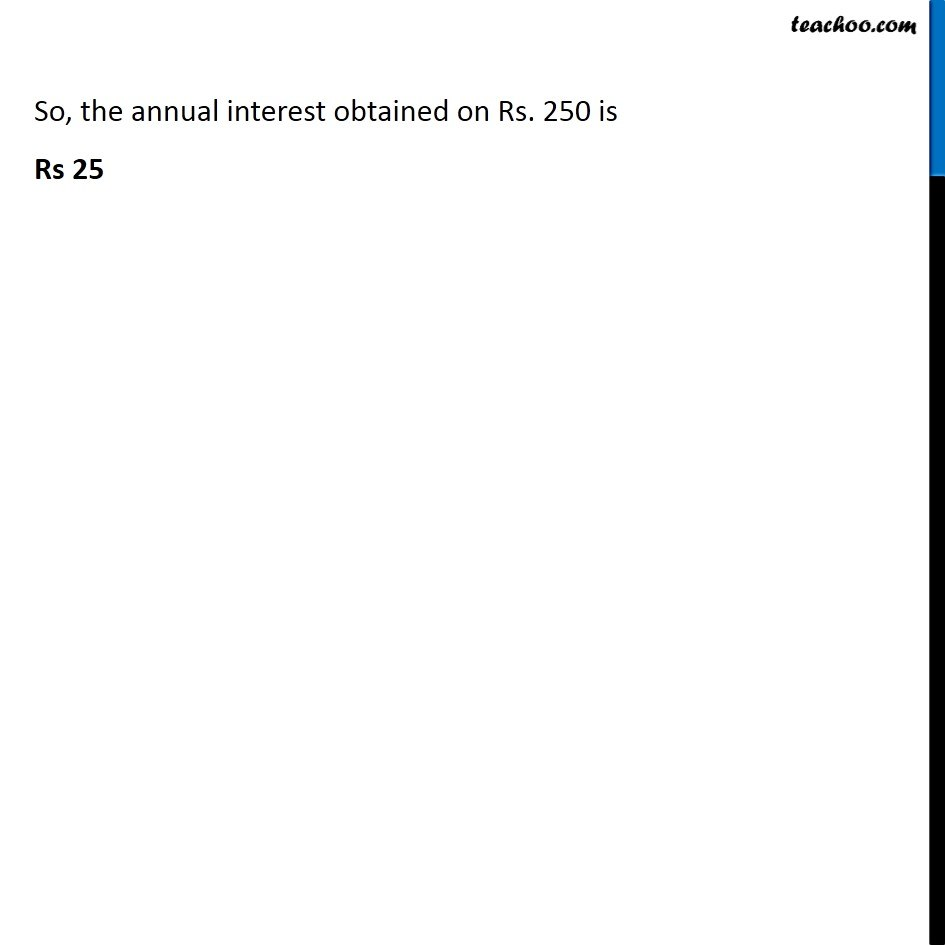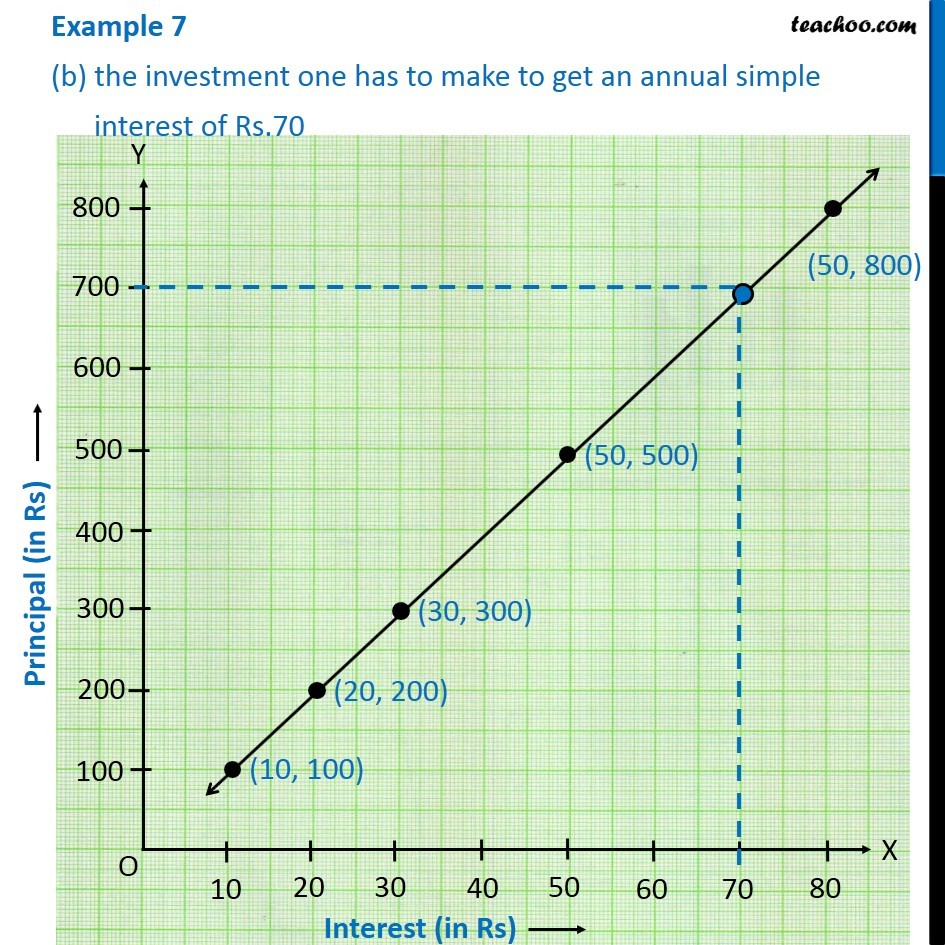Learn in your speed, with individual attention - Teachoo Maths 1-on-1 Class

### Transcript

Example 4 (Principal and Simple Interest) A bank gives 10% Simple Interest (S.I) on deposits by senior citizens. Draw a graph to illustrate the relation between the sum deposited and simple interest earned. Find from your graph. Given, Rate of Simple Interest = R = 10% The formula for simple interest is Simple Interest = (𝑃 × 𝑅 × 𝑇)/100 Here, P = Money deposited / Principal R = Rate of Interest T = Number of years We need to make a graph of sum deposited and simple interest earned So, finding different values of Interest for different Values of P Here, we take Time = 1 year Example 4 (a) the annual interest obtainable for an investment of Rs.250. So, the annual interest obtained on Rs. 250 is Rs 25 Example 4 (b) the investment one has to make to get an annual simple interest of Rs.70 So, investment made to get interest of Rs. 70 is Rs 700